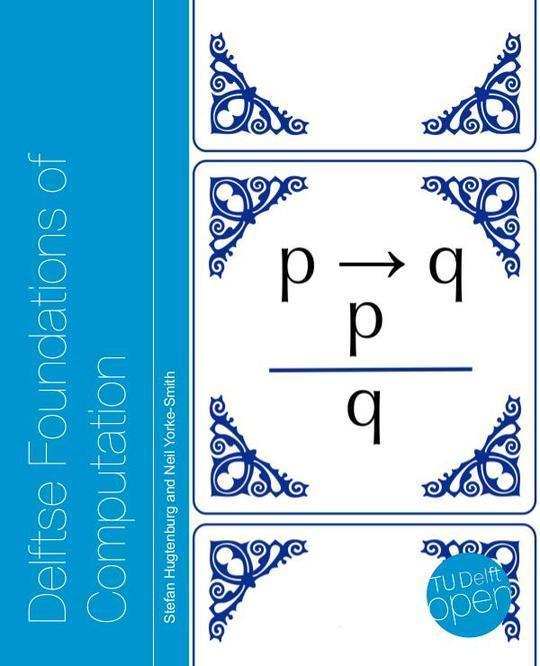Free

# 13-48-64-1-10-20181122

By Unknown
Free
Book Description
• PDF Hugtenburg & Yorke-Smith - Front
• Delftse FoC v1.0.2
• Introduction
• Logic
• Propositional Logic
• Propositions
• Logical operators
• Precedence rules
• Logical equivalence
• More logical operaters
• Implications in English
• More forms of implication
• Exclusive or
• Universal operators
• Classifying propositions
• Boolean Algebra
• Basics of Boolean Algebra
• Substitution laws
• Simplifications
• More rules of Boolean algebra
• Application: Logic Circuits
• Logic gates *
• Combining gates to create circuits *
• From circuits to propositions *
• Disjunctive Normal Form
• Predicate Logic
• Predicates
• Quantifiers
• Operators
• Tarski's world and formal structures
• Logical equivalence
• Deduction
• Arguments
• Valid arguments and proofs
• Proofs in predicate logic
• Proof
• A Little Historical Background
• Mathematical Proof
• How to write a proof
• Some terminology
• Examples
• Mathematical Induction
• How to write a proof by induction
• Examples
• More examples
• Strong Mathematical Induction
• Application: Recursion and Induction
• Recursive factorials
• Towers of Hanoi
• Binary trees *
• Recursive Definitions
• Invariants
• Sets, Functions, and Relations
• Basic Concepts
• Elements of sets
• Set-builder notation
• Operations on sets
• Visualising sets
• Sets of sets
• Mathematical induction revisited
• Structural Induction
• The Boolean Algebra of Sets
• Set complement
• Link between logic and set theory
• Application: Programming with Sets *
• Representing sets
• Computing with sets
• Functions
• Formalising the notion of functions
• Operations on functions
• Properties of functions
• First-class objects
• Application: Programming with Functions *
• Functions as first-class objects
• Counting Past Infinity
• Cardinality
• Counting to infinity
• Uncountable sets *
• A final note on infinities *
• Relations
• Properties of relations
• Equivalence relations
• Application: Relational Databases *
• Looking Beyond *